### Home > PC > Chapter 2 > Lesson 2.3.4 > Problem2-111

2-111.
1. The sum of the areas for the right-endpoint rectangles of the function A(x) =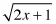using 6 rectangles on the interval 1 ≤ x ≤ 4 is (0.5)[(2 + 2.236 + … + 3)] = 7.579. Homework Help ✎

1. What does 0.5 represent?

2. Let b(x) = 2 + A(x). Use what you know to find the sum of the areas of the 6 right-endpoint rectangles of b(x) on 1 ≤ x ≤ 4.

3. Find the sum of the 6 right-endpoint rectangles for c(x) = 1000 + A(x) for 1 ≤ x ≤ 4.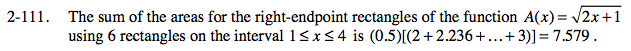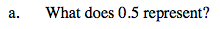Width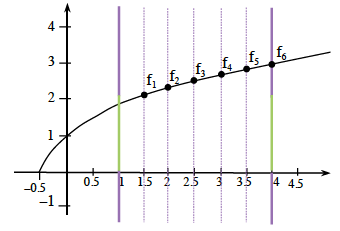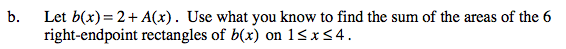The 2 in the express 2 + A(x) raises the function up 2. So the total area is increased by 6 because the base was 3.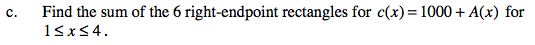Since the whole graph is raised up 1000 and the base is still 3, how much more area would the new function have than A(x)?